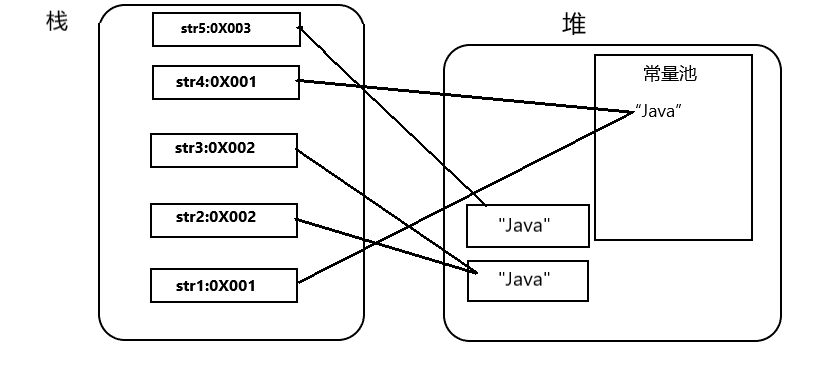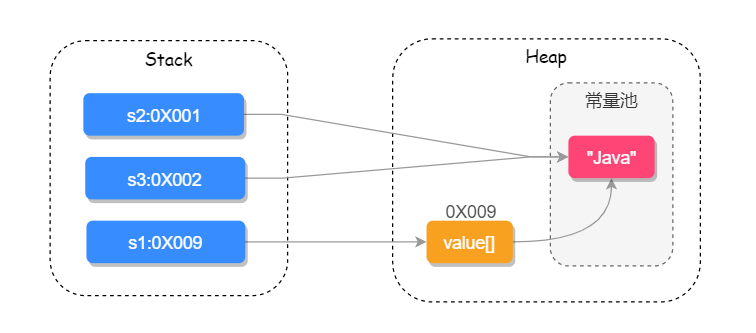## 引言

String可以说是在Java开发中必不可缺的一种类，String容易忽略的细节也很多，对String的了解程度也反映了一个Java程序员的基本功。下面就由一个面试题来引出对String的剖析。

## 1. String在源码里究竟是如何实现的，它有哪些方法，有什么作用？

``````public final class String
implements java.io.Serializable, Comparable<String>, CharSequence {
/**存储字符串的字符数组*/
private final char value[];

/** 缓存字符串的hashcode */
private int hash; // 默认是0

/** 用于验证一致性来是否进行反序列化 */
private static final long serialVersionUID = -6849794470754667710L;
``````

### 1.1 String重要构造方法

``````// String 为参数的构造方法
public String(String original) {
this.value = original.value;
this.hash = original.hash;
}
// char[] 为参数构造方法
public String(char value[]) {
//重新复制一份char数组的值和信息，保证字符串不会被修改传回
this.value = Arrays.copyOf(value, value.length);
}
// StringBuffer 为参数的构造方法
public String(StringBuffer buffer) {
synchronized(buffer) {
this.value = Arrays.copyOf(buffer.getValue(), buffer.length());
}
}
// StringBuilder 为参数的构造方法
public String(StringBuilder builder) {
this.value = Arrays.copyOf(builder.getValue(), builder.length());
}
``````

### 1.2 String重要的方法

#### 1.2.1 equals()方法

``````/**比较两个字符串是否相等，返回值为布尔类型*/
public boolean equals(Object anObject) {//比较类型可以是object
/*引用对象相同时返回true*/
if (this == anObject) {
return true;
}
/*判断引用对象是否为String类型*/
if (anObject instanceof String) { //instanceof用来判断数据类型是否一致
String anotherString = (String)anObject;
int n = value.length;
if (n == anotherString.value.length) {
//将两个比较的字符串转换成字符数组
char v1[] = value;
char v2[] = anotherString.value;
//一个一个字符进行比较
int i = 0;
while (n-- != 0) {
if (v1[i] != v2[i])
return false;
i++;
}
return true;
}
}
return false;
}
``````

`equals()`方法首先通过instanceof判断数据类型是否一致，是则进行下一步将两个字符串转换成字符数组逐一判断。最后再返回判断结果。

#### 1.2.2 compareTo()方法

``````/*比较两个字符串是否相等，返回值为int类型*/
public int compareTo(String anotherString) {//比较类型只能是String类型
int len1 = value.length;
int len2 = anotherString.value.length;
/*获得两字符串最短的字符串长度lim*/
int lim = Math.min(len1, len2);
char v1[] = value;
char v2[] = anotherString.value;
/*逐一比较两字符组的字符*/
int k = 0;
while (k < lim) {
char c1 = v1[k];
char c2 = v2[k];
//若两字符不相等，返回c1-c2
if (c1 != c2) {
return c1 - c2;
}
k++;
}
return len1 - len2;
}
``````

`compareTo()`通过逐一判断两字符串中的字符，不相等则返回两字符差，反之循环结束最后返回0

##### 小结
1. `compareTo()``equals()`都能比较两字符串，当equals()返回true，compareTo()返回0时，都表示两字符串完全相同。
2. 同时两者也有区别：
• 返回类型`compareTo()`是boolean,`equals()`是int。
• 字符类型`compareTo()`是Object,`equals()`只能是String类型。

### 1.3其他方法

1. `indexOf()`：查询字符串首次出现的下标位置
2. `lastIndexOf()`：查询字符串最后出现的下标位置
3. `contains()`：查询字符串中是否包含另一个字符串
4. `toLowerCase()`：把字符串全部转换成小写
5. `toUpperCase()`：把字符串全部转换成大写
6. `length()`：查询字符串的长度
7. `trim()`：去掉字符串首尾空格
8. `replace()`：替换字符串中的某些字符
9. `split()`：把字符串分割并返回字符串数组
10. `join()`：把字符串数组转为字符串

## 2. String常见的面试问题

### 2.1 为什么String类型要用final修饰？

• 从上面的代码可以看出，String类是被private final修饰的不可继承类。那么为何要用final修饰呢？

Java 语言之父 James Gosling 的回答是，他会更倾向于使用 final，因为它能够缓存结果，当你在传参时不需要考虑谁会修改它的值；如果是可变类的话，则有可能需要重新拷贝出来一个新值进行传参，这样在性能上就会有一定的损失。

James Gosling 还说迫使 String 类设计成不可变的另一个原因是安全，当你在调用其他方法时，比如调用一些系统级操作指令之前，可能会有一系列校验，如果是可变类的话，可能在你校验过后，它的内部的值又被改变了，这样有可能会引起严重的系统崩溃问题，这是迫使 String 类设计成不可变类的一个重要原因。

​ 所以只有当字符串不可改变时，才能利用字符常量池，保证在使用字符的时候不会被修改。

• 那么问题来了，我们在使用final修饰一个变量时，不变的是引用地址，引用地址对应的对象是可以发生变化的。如：

``````import java.util.Arrays;
public class IntTest{
public static void main(String args[]){
final char[] arr = new char[]{'a', 'b', 'c', 'd'};
System.out.println("arr的地址1：" + arr);
System.out.println("arr的值2：" + Arrays.toString(arr));
//修改arr的值
arr = 'b';
//修改arr数组的地址,这里会发生编译错误，所以无法修改引用地址
//arr = new char[]{'1', '2', '3'};
System.out.println("arr的地址2：" + arr);
System.out.println("arr的值2：" + Arrays.toString(arr));

}
}
/*运行结果：
arr的地址1：[[email protected]
arr的值1：[a b c d]
arr的地址2：[[email protected]
arr的值2：[a b b d]

显然不变的是引用地址，引用地址所指对象的内容可以被修改
*/
``````

而在上述源码中，String类下有一个私有的char数组成员

``````public final class String
implements java.io.Serializable, Comparable<String>, CharSequence   {
/**存储字符串的字符数组*/
private final char value[];
``````

那么是否可以通过修改char数组所指对象的内容，来改变string的值呢？来试一试：

``````import java.util.Arrays;
public class IntTest{
public static void main(String args[]){
char[] arr = new char[]{'a','b','c','d'};
String str = new String(arr);
System.out.println("arr的地址1:" + arr);
System.out.println("str= " + str);
System.out.println("arr[]= "+Arrays.toString(arr));
//修改arr的值
arr='b';
System.out.println("arr的地址2：" + arr);
System.out.println("str= "+str);
System.out.println("arr[]= "+Arrays.toString(arr));

}
}
/*运行结果：
arr的地址1:[[email protected]
str= abcd
arr[]= [a, b, c, d]
arr的地址2：[[email protected]
str= abcd
arr[]= [a, b, b, d]
*/
``````

显然无法修改字符串，这是为何，我们再看看构造方法

``````// String 为参数的构造方法
public String(String original) {
this.value = original.value;
this.hash = original.hash;
}
// char[] 为参数构造方法
public String(char value[]) {
//重新复制一份char数组的值和信息，保证字符串不会被修改传回
this.value = Arrays.copyOf(value, value.length);
}
``````

发现string的构造方法里将原来的char数组的值和信息copy了一份，保证字符串不会被修改传回。

### 2.2 equals()和 == 的区别

#### 2.2.1 先说结论：

• ==在基本类型中比较其对应的值，在引用类型中比较其地址值

• equals()在未被重写时和 == 完全一致，被重写后是比较字符串的值

``````public class StringTest {
public static void main(String args[]) {
String str1 = "Java"; //放在常量池中
String str2 = new String("Java"); //在堆中创建对象str2的引用
String str3 = str2; //指向堆中的str2的对象的引用
String str4 = "Java"; //从常量池中查找
String str5 = new String("Java");
System.out.println(str1 == str2); //false
System.out.println(str1 == str3); //false
System.out.println(str1 == str4); //true
System.out.println(str2 == str3); //true
System.out.println(str2 == str5); //false
System.out.println(str1.equals(str2)); //true
System.out.println(str1.equals(str3)); //true
System.out.println(str1.equals(str4)); //true
System.out.println(str2.equals(str3)); //true
}
}
``````

实际上`equals()`方法也是继承Object的`equals()`方法。

``````public boolean equals(Object obj) {
return (this == obj);
}
``````

从上面的`equals()`方法的源码可以看出，String在继承方法后对应修改了方法中的相关内容，所以上述代码的`equals()`方法输出都是true。

​ 类似于`String str1 = "Java"; ` 的和`String str2 = new String("Java");`形式有很大的区别，`String str1 = "Java"; `形式首先在编译过程中Java虚拟机就会去常量池中查找是否存在“Java”,如果存在，就会在栈内存中开辟一块地方用于存储其常量池中的地址。所以这种形式有可能创建了一个对象（常量池中），也可能一个对象也没创建，即str1是直接在常量池中创建“Java”字符串，str4是先在常量池中查找有“Java”,所以直接地址直接指向常量池中已经存在的”Java“字符串。

`String str2 = new String("Java");`的形式在编译过程中，先去常量池中查找是否有“Java”,没有则在常量池中新建”Java”。到了运行期，不管常量池中是否有“Java”，一律重新在堆中创建一个新的对象，然如果常量池中存在“Java”,复制一份放在堆中新开辟的空间中。如果不存在则会在常量池中创建一个“Java”后再复制到堆中。所以这种形式至少创建了一个对象，最多两个对象。因此str1和str2的引用地址必然不相同。### 2.3 string中的intern()方法

​ 调用intern方法时，如果常量池中存在该字符串，则返回池中的字符串。否则将此字符串对象添加到常量池中，并返回该字符串的引用。

``````String s1 = new String("Java");
String s2 = s1.intern();//直接指向常量池中的字符串
String s3 = "Java";
System.out.println(s1 == s2); // false
System.out.println(s2 == s3); // true
``````### 2.4 String和StringBuilder、StringBuffer的区别

​ 关于这三者的区别，主要借鉴这篇博文String,StringBuffer与StringBuilder的区别??首先，String是字符串常量，后两者是字符串变量。其中StringBuffer是线程安全的，下面说说他们的具体区别。

​ String适用于字符串不可变的情况，因为在经常改变字符串的情形下，每次改变都会在堆内存中新建对象，会造成 JVM GC的工作负担，因此在这种情形下，需要使用字符串变量。

​ 再说StringBuffer,它是线程安全的可变字符序列，它提供了append和insert方法用于字符串拼接，并用synchronized来保证线程安全。并且可以对这些方法进行同步，像以串行顺序发生，而且该顺序与所涉及的每个线程进行的方法调用顺序一致。

``````@Override
public synchronized StringBuffer append(Object obj) {
toStringCache = null;
super.append(String.valueOf(obj));
return this;
}

@Override
public synchronized StringBuffer append(String str) {
toStringCache = null;
super.append(str);
return this;
}

``````

​ 最后是StringBuilder,因为StringBuffer要保证线程安全，所以性能不是很高，于是在JDK1.5后引入了StringBuilder,在没有了synchronize后性能得到提高，而且两者的方法基本相同。所以在非并发操作下，如单线程情况可以使用StringBuilder来对字符串进行修改。

### 2.5 String中的“ + ”操作符

​ 其实在2.4中提到，String是字符串常量，具有不可变性。所以在拼接字符串、修改字符串时，尽量选择StringBuilder和StringBuffer。下面再谈一谈String中出现“+”操作符的情况：

``````String s1 = "Ja";
String s2 = "va";
String s3 = "Java";
String s4 = "Ja" + "va"; //在编译时期就在常量池中创建
String s5 = s1 + s2; //实际上s5是stringBuider,这个过程是stringBuilder的append

System.out.println("s3 == s4 " + (s3 == s4));
System.out.println("s3 == s5 " + (s3 == s5));

/**

s3 == s4 true
s3 == s5 false
*/
``````

``````1  String s = "Ja";//s1
2  String s1 = "va";//s2
3  String s2 = "Java";//s3
4  String s3 = "Java";//s4
5  String s4 = (new StringBuilder()).append(s).append(s1).toString();//s5
6  System.out.println((new StringBuilder()).append("s3 == s4").append(s2 == s3).toString());
7  System.out.println((new StringBuilder()).append("s3 == s5").append(s2 == s4).toString());
``````